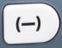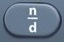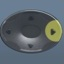# SquaringNumber Squared = Number × Itself = Number²

Basics

Number Squared = Number × Itself  = Number²

3 squared = 3 × 3 = 3² = 9
12 squared = 12 x 12 = 12² = 144
20 squared = 20 × 20 = 20² = 400

In order to get the correct answer when squaring a negative number, place the negative number in parentheses to protect the order of operations.
Right:  -3 squared = (-3)² = 9
Wrong:  -3 squared = -3² = -9is the squaring command.

It is possible that questions involving squaring will be in the calculator-prohibited section of the test.  In that case, it is helpful to know your multiplication and division tables.

Formulas (NOT on Mathematics Formula Sheet)

Number Squared = Number × Itself = Number²

Question

What is 4²?

16

Input Display Commentblinker clears screen4 squared16 Answer
###### Number²

Question
According to the calculator, what is the value of -4²?
According to the calculator, what is the value of (-4)²
?
Observe how parentheses change the results of these calculations.

-4² = -16

(-4)² = 16

The second calculation illustrates the way parentheses protect the order of operations when squaring a negative number.

Input Display Commentblinker clears screen4-4²-16blinker clears screen4(-4)²
###### Parentheses protect order of operations.16
###### Number²

Question

What is$\bf\displaystyle\frac{1}{4}$ squared?$\bf\displaystyle\frac{1}{16}$

Input Display Commentblinker clears screen14$\bf\displaystyle{{\frac{1}{4}}^{2}}$$\bf\displaystyle\frac{1}{4}$ squared$\bf\displaystyle\frac{1}{16}$ Answer
###### Number²

Question
What is 71.4 squared?

5097.96

Input Display Commentblinker clears screen
71.471.4² 71.4 squared5097.96 Answer
###### Number²

Question
Without using the calculator, what is 5 squared?

25

5 squared = 5² = 5 × 5 = 25

Question
Without using the calculator, what is -5 squared?

25

-5 squared = (-5)² = -5 × -5 = 25

Practice – Questions

1.  What is 9²?

2.  What is 9 squared?

3.  What is (-9)²?

4.  What is -9 squared?

5.  What is -9²?

6.  What is$\bf\displaystyle\frac{1}{2}$ squared?

7.  What is$\bf\displaystyle\frac{3}{4}$ squared?

8.  What is 313.25 squared?

9.  Without using the calculator, what is 7 squared?

10.  Without using the calculator, what is -7 squared?

1.  81

2.  81

3.  81

4.  81

5.  -81

6.$\bf\displaystyle\frac{1}{4}$

7.$\bf\displaystyle\frac{9}{16}$

8.  98125.5625

9.  49

10.  49